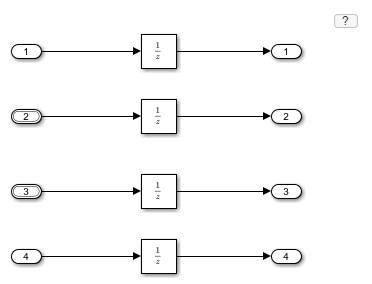# createInputDataset

Generate dataset object for root-level Inport or bus element ports in model

## Syntax

``[inports_dataset] = createInputDataset(model)``
``[inports_dataset] = createInputDataset(model,'DatasetSignalFormat',value)``

## Description

example

````[inports_dataset] = createInputDataset(model)` generates a `Simulink.SimulationData.Dataset` object from the root-level Inport blocks or bus element ports in a model. Signals in the generated dataset have the properties of the root inports and the corresponding ground values at model start and stop times. You can create `timetable` or `timeseries` objects for the time and values for signals for which you want to load data for simulation. The other signals use ground values.`[inports_dataset] = createInputDataset(model,'DatasetSignalFormat',value)` generates a `Simulink.SimulationData.Dataset` object whose signal dataset signal elements are either `timeseries` or `timetable`.```

## Examples

collapse all

This example shows how to create a timeseries dataset with elements for the four root-level Inport blocks in a model. Use that dataset as a basis for creating a dataset to load signal data into the model.

The In1 block outputs a double, `In2` and `In3` each output a nonvirtual bus, and `In4` outputs an `int16`.

```mdl = 'ex_dataset_for_inports'; open_system(mdl) ```Create a `Dataset` object for the root-level `Inport` blocks.

```ds = createInputDataset(mdl) ```
```Exporting logged dataset prior to deleting run...done. ds = Simulink.SimulationData.Dataset '' with 4 elements Name BlockPath ____ _________ 1 [1x1 timeseries] In1 '' 2 [1x1 struct ] In2 '' 3 [1x1 struct ] In3 '' 4 [1x1 timeseries] In4 '' - Use braces { } to access, modify, or add elements using index. ```

Replace the placeholder value for the first signal in the `Dataset` with actual signal values that you want to load into the model.

```ds{1} = ds{1}.delsample('Index',[1,2]); ds{1} = ds{1}.addsample('time',[1 3 3 10]','data',[1 1 5 5]'); ```

Examine the `In2` signal.

```ds{2} ```
```ans = struct with fields: a: [1x1 timeseries] b: [1x1 timeseries] ```

For `In2` , create data for bus elements `a` and `b`.

```ds{2}.a = ds{2}.a.delsample('Index',[1,2]); ds{2}.a = addsample(ds{2}.a,'time',[1:10]','data',[1:10]'); ds{2}.b = timeseries((1:10)',0.1:.1:1,'Name','sig2_b'); ```

For `In3`, specify data for element `a` of the bus, and use ground values for element `b`.

```ds{3}.a = timeseries((1:10)',0.1:.1:1,'Name','sig3_a'); ```

Plot `ds`.

```plot(ds) ```

Set the Input configuration parameter to `ds`. Alternatively, you can use the Root Inport Mapper tool to set the Input parameter.

```set_param(mdl,'LoadExternalInput','on'); set_param(mdl,'ExternalInput','ds'); ```

Run the simulation. The `Inport` blocks use the signal data specified in `ds` or ground values for elements that do not have specified signal data.

```sim(mdl) ```

## Input Arguments

collapse all

Model for which to generate a dataset with an element for each root-level Inport block, specified as a string, character vector, or model handle.

Signal format for dataset signal elements, specified as `'timetable'` or `'timeseries'`.

## Output Arguments

collapse all

Dataset with an element for each root-level Inport block, returned as a `Simulink.SimulationData.Dataset` object.

## Version History

Introduced in R2017a

expand all